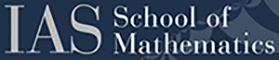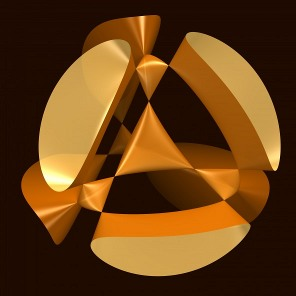### Highlights#### The Topology of Algebraic Varieties

IAS - September 2015During the academic year 2014-2015, the School of Mathematics conducted a special program on The Topology of Algebraic Varieties. The program was led by Distinguished Visiting Professor Claire Voisin , from CNRS and Institut de Mathématiques de Jussieu, and Member Burt Totaro from UCLA. The central themes of the program were Hodge theory and algebraic cycles. A large group of Members took part in the program. The senior Members who participated for the full year included Francis Brown, Institut de Mathématiques de Jussieu; Richard Hain, Duke University; Matthew Kerr, Washington University at St. Louis; Bruno Klingler, Institut de Mathématiques de Jussieu; Janos Kollar, Princeton University; Radu Laza, Stony Brook University; and Fabien Morel, University of Munich.

Background and Motivation.

Algebraic Geometry is the study of spaces (called algebraic varieties) de ned by polynomial equations, called algebraic varieties. When the eld of coecients is the real or complex numbers, an algebraic variety can typically be viewed as a manifold, and it has topological invariants such as singular cohomology and the fundamental group. One great success of algebraic geometry is Alexander Grothendieck’s theory of etale cohomology and the etale fundamental group, which produces similar computable invariants in a purely algebraic way, for varieties over any eld.

On the other hand, one central goal of algebraic geometry which remains mysterious is to describe all the subvarieties of a given algebraic variety. More precisely, one considers algebraic cycles , which are linear combinations of subvarieties, and Chow groups , which are the algebraic cycles modulo a natural equivalence relation. We want to understand the Chow groups of an algebraic variety in terms of its Hodge theory , which is a more computable invariant related to singular cohomology and integrals of algebraic functions (known as periods). The Hodge conjecture is a model problem of this type.

The Chow groups of codimension-one cycles has been related to the theory of Hodge
structures of weight 1 since the 19th century (Abel’s theorem), and there are now major open conjectures (Bloch, Bloch-Beilinson) relating the size of Chow groups in any codimension to the size of Hodge structures. These conjectures t well with the generalized Hodge conjecture due to Grothendieck, which concerns the codimension of support of a Hodge structure.

Another distinctive feature of algebraic geometry is that the space of algebraic varieties of a given type can itself be viewed as an algebraic variety, known as a moduli space . There are deep interactions between moduli spaces and the theory of algebraic cycles. For example, Professor Pierre Deligne’s notion of an absolute Hodge class depends on the possibility of changing the coecients of the de ning equations. Voisin made progress on the Bloch-Beilinson conjecture for complete intersection varieties by considering the moduli space of all such varieties. Over the complex numbers, the study of the deformations of a variety leads to the theory of periods and associated monodromy representations. Over number elds, this is replaced by the notion of Galois representations.

The interaction of people working on the topology of algebraic varieties from these many di erent viewpoints made this one-year program very fruitful.

The program had four main themes:

1. Topological restrictions on complex projective manifolds . This includes restrictions on fundamental groups, and an essential tool is Hodge theory and the study of the moduli spaces of representations of the fundamental group (non-abelian Hodge theory). The analysis of the fundamental group and its pro nite completion also has deep arithmetic aspects that have been studied by Members Hain and Brown.
2. Moduli spaces: construction and properties . This subject is very much related to the previous one via the period map and the theory of variations of Hodge structures, which is a fundamental tool developed by Professor Phillip Griths to study both the topology or motive of a given variety and the properties of moduli spaces. Moduli spaces usually have a rich fundamental group represented on cohomology (monodromy representation) and this conversely has strong consequences on their topology and curvature properties. The program included an informal working group on moduli spaces and the minimal model program.
3. Motives and Chow groups . Chow groups are not well understood but they have properties which make them very useful and easy to manipulate, like the “localization exact sequence.” The general expectation (summarized under the name of the Bloch-Beilinson conjectures) is that over the complex numbers, Chow groups are governed in a rather precise way by the shape of the Hodge structures. The Chow groups also provide the most re ned theory to speak about motives, the motive being the study of algebro-geometric properties of a variety that can be re ected on cohomology via the cycle class map. The Chow ring is also a very rich object with very distinctive properties (partially conjectural) in the case of hyperkahler manifolds. The program included an informal working group on Chow groups and their relation to Hodge theory.
4. Singularity theory . This is classically a very important part of the study of the topology of algebraic varieties over the complex numbers, that is, in the analytic setting. A crucial tool in the study of singular varieties is mixed Hodge theory, as developed by Professor Pierre Deligne. This has become still more important with the recent progress of birational geometry, which produces minimal models which are in general singular.

Here are some of the numerous advances achieved during the program:

1. Junyi Xie and Member Serge Cantat proved the rst signi cant restrictions on the birational automorphism group of an arbitrary algebraic variety. For example, the group SL(n, Z ) cannot act faithfully by birational transformations on a variety of dimension less than n — 1.
2. Members Janos Kollar and Chenyang Xu proved that the dual complex of a Calabi-Yau pair in low dimensions is homeomorphic to a nite quotient of a sphere. This statement was expected as part of mirror symmetry, the family of algebro-geometric conjectures inspired by string theory.
3. Voisin introduced a new approach to a central problem of algebraic geometry: which varieties are rational or stably rational? Her method, an unexpected application of the theory of algebraic cycles, has led to a series of rapid advances. In particular, Totaro proved that most hypersurfaces of degree at least about two-thirds their dimension are not stably rational.
4. Hacon, McKernan, and Member Chenyang Xu made a fundamental contribution to the construction of a compact moduli space for stable varieties of general type, showing that the stable varieties of given degree form a bounded family.

In addition to numerous one-hour lectures, the program included several three-hour
lecture series, for example by Francis Brown (The projective line minus 3 points), Serge Cantat (Birational actions of SL(n, Z )), Fabien Morel (On the homology and the tree of SL(2) over polynomial rings), Bruno Klingler (André-Oort conjecture), and Chenyang Xu (Boundedness of log general type pairs).

Workshops:

Two workshops were organized as part of the program, with outstanding speakers from the Institute and beyond. The rst workshop was on Fundamental groups and periods . Several speakers used analytic methods to give powerful restrictions on the possible fundamental groups of smooth projective varieties. Arithmetic aspects of fundamental groups were also presented: representations of étale fundamental groups and at connections in nonzero characteristic, the fundamental group of the projective line minus 3 points, mixed Tate motives and multizeta values.

The second workshop was on Chow groups, motives and derived categories . The notion of the derived category of an algebraic variety has been studied intensely, as a precise and refined way of studying a variety starting from the study of its vector bundles. The last point is also the starting point of algebraic K-theory and the closely related Chow groups. The derived category itself contains much more information. Thus di erent aspects of algebraic K-theory and its recent developments in various guises were represented. Some talks also addressed Chow groups over non-algebraically closed elds and applications to rationality questions.

As a complement to these workshops, a conference on rationality problems was organized at Stony Brook University by R. Laza, C. Schnell, J. Starr and C. Voisin, focusing on the applications of some of the tools sketched above to the so-called Lüroth problem, or its stable version, searching for criteria characterizing rational or stably rational varieties. Two recently developed new tools or approaches for the Luroth problem were discussed and compared: methods of algebraic cycles (decomposition of the diagonal) and methods of derived categories.

Finally, two special days on algebraic geometry were organized, one joint with Columbia University and one with Princeton University.

** Image by Oliver Labs